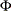### Functional Summary

The statements and options used with the PANEL procedure are summarized in the following table.

Description

Statement

Option

Data Set Options

Includes correlations in the OUTEST= data set

PANEL

CORROUT

Includes covariances in the OUTEST= data set

PANEL

COVOUT

Specifies the input data set

PANEL

DATA=

Specifies variables to keep but not transform

FLATDATA

KEEP=

Specifies the output data set for CLASS STATEMENT

CLASS

OUT =

Specifies the output data set

FLATDATA

OUT =

Specifies the name of an output SAS data set

OUTPUT

OUT=

Writes parameter estimates to an output data set

PANEL

OUTEST=

Writes the transformed series to an output data set

PANEL

OUTTRANS=

Requests that the procedure produce graphics via the Output Delivery System

PANEL

PLOTS=

Declaring the Role of Variables

Specifies BY-group processing

BY

Specifies the classification variables

CLASS

Transfers the data into uncompressed form

FLATDATA

Specifies the cross section and time ID variables

ID

Declares instrumental variables

INSTRUMENTS

Lag Generation

Specifies output data set for lags where missing values are replaced with the cross section mean

CLAG

OUT=

Specifies output data set for lags with missing values included

LAG

OUT=

Specifies output data set for lags where missing values are replaced with the time period mean

SLAG

OUT=

Specifies output data set for lags where missing values are replaced with overall mean

XLAG

OUT=

Specifies output data set for lags where missing values are replaced with zero

ZLAG

OUT=

Printing Control Options

Prints correlations of the estimates

MODEL

CORRB

Prints covariances of the estimates

MODEL

COVB

Suppresses printed output

MODEL

NOPRINT

Requests that the procedure produce graphics via the Output Delivery System

MODEL

PLOTS=

Prints fixed effects

MODEL

PRINTFIXED

Performs tests of linear hypotheses

TEST

Model Estimation Options

Requests the Breusch-Pagan test for one-way random effects

MODEL

BP

Requests the Breusch-Pagan test for two-way random effects

MODEL

BP2

Specifies the between-groups model

MODEL

BTWNG

Specifies the between-time-periods model

MODEL

BTWNT

Requests the clustered HCCME estimator for the variance-covariance matrix

MODEL

CLUSTER

Specifies the Da Silva method

MODEL

DASILVA

Specifies the one-way fixed-effects model

MODEL

FIXONE

Specifies the one-way fixed-effects model with respect to time

MODEL

FIXONETIME

Specifies the two-way fixed-effects model

MODEL

FIXTWO

Specifies the Moore-Penrose generalized inverse

MODEL

GINV = G4

Specifies the dynamic panel estimator model

MODEL

GMM

Requests the HAC estimator for the variance-covariance matrix

MODEL

HAC=

Requests the HCCME estimator for the variance-covariance matrix

MODEL

HCCME=

Specifies the order of the moving average error process for Da Silva method

MODEL

M=

Suppresses the intercept term

MODEL

NOINT

Specifies the Parks method

MODEL

PARKS

Prints thematrix for Parks method

MODEL

PHI

Specifies the pooled model

MODEL

POOLED

Requests poolability tests for one-way fixed effects and pooled model

MODEL

POOLTEST

Specifies the one-way random-effects model

MODEL

RANONE

Specifies the two-way random-effects model

MODEL

RANTWO

Prints autocorrelation coefficients for Parks method

MODEL

RHO

Controls the check for singularity

MODEL

SINGULAR=

Specifies the method for panel unit root/stationarity test

MODEL

UROOTTEST=

Specifies the method for the variance components estimator

MODEL

VCOMP=

Specifies linear equality restrictions on the parameters

RESTRICT

Specifies the TEST statement

TEST

WALD, LM, LR